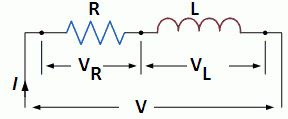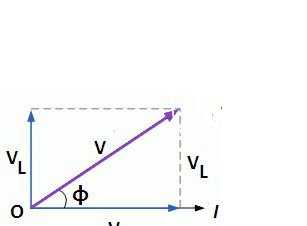# What is RL Series Circuit? – Phasor Diagram & Power Curve

0
503

## What is RL Series Circuit?

#### A RL series circuit having resistance R and inductance L is shown in the figure below.RL SERIES CIRCUIT

#### Let, V = RMS value of applied voltage. I = RMS value of currentVr = Voltage drop across resistance = I*RVₗ = Voltage drop across inductance =  I*XₗWe know that current in the pure inductance circuit lags behind the voltage by 90 degree. Therefore, Voltage drop across inductance, Vₗ is ahead of I by 90 degree. Phasor diagram of series RL circuit is shown in figure. * Voltage drop across R is in phase with the current. * Voltage drop across L leads current by 90.PHASOR DIAGRAM OF RL SERIES CIRCUIT